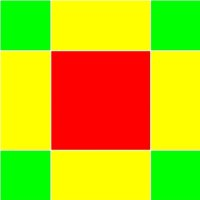# Completion of Squares

## Exhibit: table of content

##### Oliver Knill, SciCtr 434, knill@math.harvard.eduThe "completion of squares" is used to find solutions to quadratic equations. In multivariable calculus, you use it to find the center of circles or spheres or more generally to understand quadrics, surfaces of the form g(x,y,z)=0 where g is quadratic.

The idea is one of the oldest ideas in Mathematics. The mathematician Al Khwarizmi, used both algebraic and geometric methods to solve quadratic equations writes for example to describe the solution of
x2 + 10 x = 39:
 ``` x2 + b x + c = 0 x2 + b x + b2/4 = b2/4 -c (x+b/2)2 = b2/4 -c x = (b2/4 -c)1/2 - b/2 ```"... a square and 10 roots are equal to 39 units. The question therefore in this type of equation is about as follows: what is the square which combined with ten of its roots will give a sum total of 39? The manner of solving this type of equation is to take one-half of the roots just mentioned. Now the roots in the problem before us are 10. Therefore take 5, which multiplied by itself gives 25, an amount which you add to 39 giving 64. Having taken then the square root of this which is 8, subtract from it half the roots, 5 leaving 3. The number three therefore represents one root of this square, which itself, of course is 9. Nine therefore gives the square.The picture to the left had been drawn by Al Khwarzimi and it gives a geometric meaning to the expression "completion of squares". If we want to write x2 + 4 b x as a complete square, we interpret x2 as a square and 4 b x as 4 rectangles adjoint to the square. To have a full square, add 4 squares of length b: x2 + 4 b x + 4 b2 = (4+2b)2.

Note that Al Khwarzimi, the father of algebra, did not yet use any variables like "x" to derive the solutions of quadratic equations. He adds 25 to both sides of the equation x2 + 10 x = 39 to get (x+5)2 = 82 so that x=3.